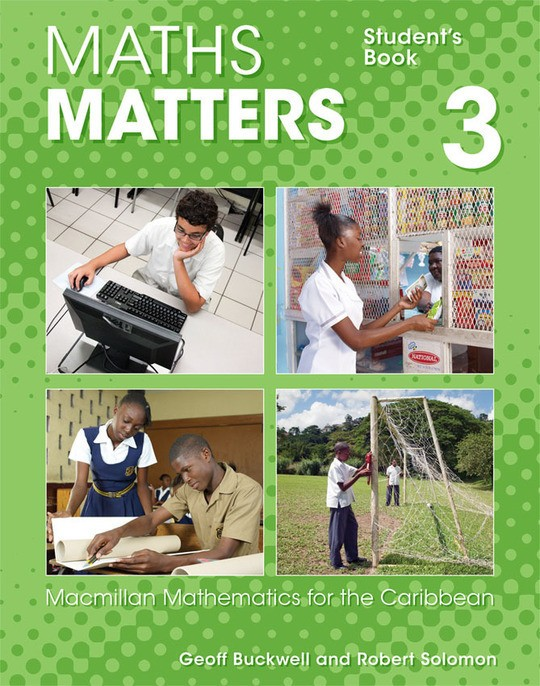\$16.85

# Maths Matters Student's Book 3

By Geoff Buckwell
US\$ 16.85
The publisher has enabled DRM protection, which means that you need to use the BookFusion iOS, Android or Web app to read this eBook. This eBook cannot be used outside of the BookFusion platform.
Book Description

• Cover
• Title
• Contents
• Introduction
• Scope and sequence
• 1 Sets
• 1.1 Revision of set notation
• 1.2 Revision of problems with two sets
• 1.3 Problems with three sets
• 2 More numbers and decimals
• 2.1 Revision of decimals
• 2.2 Significant figures
• 2.3 Estimation
• 2.4 Powers and indices
• 2.5 Standard form or scientific notation for large numbers
• 3 Algebra I (expressions)
• 3.1 Revision of terms
• 3.2 Expanding brackets
• 3.3 Indices in algebra
• 3.4 Highest common factor
• 3.5 Factorising expressions
• 4 Consumer arithmetic
• 4.1 Unit costs and best buys
• 4.2 Hire purchase
• 4.3 Commission
• 4.4 Foreign currencies
• 5.1 Classification of types of quadrilateral
• 5.2 Angles in a quadrilateral
• 5.3 Area of special quadrilaterals
• 5.4 Estimating
• 5.5 Irregular shapes
• 5.6 Sectors of a circle
• 6 Ratio and proportion
• 6.1 Ratio
• 6.2 Proportion
• 7 Enlargement
• 7.1 Enlargement
• 7.2 Scale diagrams
• 8 Algebra II (equations)
• 8.1 Equations
• 8.2 Transformation of formulae
• 8.3 Simultaneous equations
• 9 Inequalities
• 9.1 Writing statements with inequalities
• 9.2 Linear inequalities
• 9.3 Inequalities on the number line
• 9.4 Regions
• 10 Geometrical constructions
• 10.1 Constructions and drawings
• 10.2 Constructing perpendicular lines
• 10.3 Constructing parallel lines
• 10.4 Bisecting an angle
• 10.5 Bisecting a line
• 10.6 Constructing special angles
• 10.7 Constructing triangles
• 11 Solids
• 11.1 Sketching solids
• 11.2 Plan and elevation
• 11.3 Surface area
• 11.4 Volume of a prism
• 12 Pythagoras' theorem
• 12.1 The theorem
• 12.2 Finding sides using the theorem
• 12.3 Algebraic proof
• 13 Statistics and probability
• 13.1 Graphs, tables and charts
• 13.2 Mean, median and mode from frequency tables
• 13.3 Probability
• 14 Trigonometry
• 14.1 The tangent ratio
• 14.2 The sine ratio
• 14.3 The cosine ratio
• 14.4 Bearings
• 14.5 Solving triangles
• 15 Quadratic mappings and graphs
• 16 Computers
• 16.1 Calculators and computers
• 16.2 Parts of a computer (hardware)
• 16.3 Uses of a computer (software)
• 16.4 Information communication technology
• Homework and practice exercises
• Glossary
• Index
• A
• B
• C
• D
• E
• F
• G
• G
• I
• M
• N
• O
• P
• Q
• R
• S
• T
• U
• V
• Z
• Backcover
The book hasn't received reviews yet.
You May Also Like